# Time for AI: Types of Machine Learning – What’s Going on Behind the Scenes, Shown With Examples from Synerise Solutions

Our AI-driven solutions will uplift your revenue, shorten the client’s path to conversion and improve overall client satisfaction.

All this is enclosed in the automated marketing processes we offer. So why not sit back with a coffee and find out how our products work. Let’s take a dive into machine learning!Junior Solution Architect

## Let’s start with a reminder of the definition of machine learning

As Tom M. Mitchell, University Professor at the Carnegie Mellon University, said: machine learning is “a computer program is said to learn from experience E with respect to some class of tasks T and performance measure P if its performance at tasks in T, as measured by P, improves with experience E.”

In other words, the program is performing a task (T), whether it’s playing a game of chess, recognizing speech or identifying kittens in a picture. While doing this, it is gaining experience (E). To find out how good the program is at performing its task, we use a performance measure (P), the probability of winning the game of chess, the number of correctly recognized words or identified kittens.

In other words, the program is learning to do its task, and we measure how well it’s going with certain metrics.

## Types of Machine Learning:

We can distinguish 3 types of machine learning:

1.    Supervised Learning
2.    Unsupervised Learning
3.    Reinforcement Learning

Let's take a closer look at what are the differences between them.### 1.    Supervised Learning

Supervised learning can be compared to learning with a teacher. When the student is performing a task, the teacher is the judge of how well it is done. For an algorithm to learn under supervision, a labeled dataset is required. This means that the dataset contains the answers to the task that the program will perform. First, the algorithm will train on part of the dataset, learning the features connected to the tags. Then on the test part of the dataset we will measure its performance in predicting values.

Supervised learning is mainly used in two areas: classification and regression problems. If the algorithm is used for a classification problem, we expect the output to be a discrete value, assigning the input data into a group or class. However, if it is used for a regression problem, the continuous input is mapped to a continuous output.

Where does Synerise use supervised learning?

We use supervised learning in our Churn Analytics solution. Churn analysis is a very useful tool, described in another one of our blogposts. It works based on a list of a client’s features, like days since first transaction, total transaction amount, days between transactions, and labels: whether or not the customer churned, and if so – when? Learning based on the labels, and assigning importance to features, the models are capable of learning to predict the probability of a given customer churning in a given time period.

Our models are capable of discovering feature importance. Based on customer behavior, we can predict which features have a higher impact on a customer’s potential to churn based on the calculated SHAP value. Cohort analysis, on the other hand, allows us to predict the probability of a customer churning in each period of time, based on his or her actions, or lack thereof.

How can we control the performance of a supervised learning model?

One way to control a regression model is to compute the mean squared error. To calculate it we use the following formula: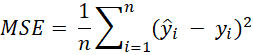where n is the number of observations,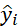is the predicted value and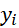is the actual value. For a better understanding, we can also use the RMSE (Root Mean Squared Error). It has the same units as our data, which makes the results easier to interpret.

To control a classification model, we use a confusion matrix, it is shown below:Based on the confusion matrix we can calculate measures:

Accuracy =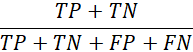is the number of correctly classified observations.
One must be cautious using this measure. If one class is much bigger than the other, we might have a false sense that our model is performing well.

True Positive Rate =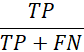,is a metric showing how well our model prevents giving false negative predictions.
Also known as Sensitivityor Recall.

True Negative Rate =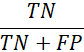is a metric showing how well out model prevents giving false positive predictions.
Also known as Specificity or Selectivity.

False Positive Rate =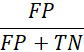also called the false alarm rate, lets us know how often we classify an observation as positive when in reality it’s negative.

Precision =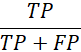measures how many of the classifications that the model “thinks” are true are actually true.
Also known as Positive Predictive Value.

F1 =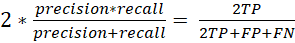,  is a harmonic mean of the precision and recall.It takes values between 0 (worst value) and 1 (perfect precision and recall).

If the output of the classifier is a numeric probability, as opposed to class labels then we can use the Log-Loss. It is an accuracy measure considering probabilistic confidence. The goal of the model is to minimize this metric, and it takes values between 0 and 1.

The mathematical formula, for a binary classification problem, is given below:

log-loss =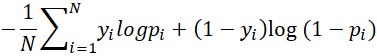, where:

y – a binary indicator of whether the class label is the correct classification
p – the model’s predicted probability that the i'th observation is of the predicted class

It is also possible to plot the performance of machine learning models.

The first example of such a plot is the PR curve. It is the curve between precision and recall for various threshold values. The better models are those which achieve high precision and recall values.Another example of a useful graph is the ROC curve. ROC stands for Receiver Operating Characteristic.

The graph is the True Positive Rate plotted against the False Positive Rate.
The better model will have a higher True Positive Rate, while maintaining a low False Positive Rate.In both above cases the graphs can be hard to compare.
That is why we can measure the Area Under the Curve, AUC. The curve with a higher area under it represents the better model.

### 2.    Unsupervised Learning

Unsupervised learning comes in handy when we do not have a labeled dataset, or we are giving the algorithm a task we do not even know the answer to. The model is given this dataset, without labels or a research question and tries to find some structure in the data on its own.

Unsupervised learning can be used for clustering, anomaly detection and association.

Where does Synerise use unsupervised learning?

We use unsupervised learning for our recommendation engine. Gathering data from a wide range of sources, beginning from features of the products used for similar recommendations to user generated data for personalized recommendations, as well as up- and cross-selling. Another example of recommendations generated from our recommendation engine are personalized promotions, where we combine the behavioral data of the clients with the features of the products.

All these features and behavioral data, recorded in events, are embedded into vectors, and the models find similarities between products of associations in purchased items. The process is unsupervised, because we don’t have the answer to the question, for example, of what to recommend to a customer who bought products X and Y but also viewed product Z. We depend on the model finding structure in the data that we feed it.
Because there is a lack of labeled data it is impossible to measure the performance of the model with the metrics described for supervised machine learning models.

However, we can measure the performance on a higher level, controlling the conversion of customers, or revenue generated thanks to our models. That is one of the reasons A/B testing is a very useful feature.

### 3.    Reinforcement Learning

In this type of machine learning there is a particular goal set. The models try to find a way to accomplish this goal in the most optimal way. The goal may be to reach some score or improve performance on some task, however the target labels are not given.

At first the model takes random actions. Anytime the algorithm conducts an action that brings it closer to the goal it receives a reward. To make the next step it relies on previous feedback as well as trying out new tactics. This also means that the model has to think about the long-term effects of its actions.

Where does Synerise use reinforcement learning?

We use reinforcement learning in automated A/B/X tests. The A/B/X tests allow us to test two or more versions of a recommendation campaign. We can also test campaigns against each other or test a campaign with a control group not taking part in the campaign.

At Synerise we allow for an automation of the test. Instead of conducting a test for a period of time and then comparing the results, the test will automatically increase the percent of audience for the version that is converting better.

The goal here is to generate higher conversion, whether it is revenue or CTR. Generating higher values in those metrics grants the model a reward. In the case of automated A/B/X testing this will mean that the model will display the best campaign to an increasingly higher percentage of the audience, consisting of your customers.

## Conclusions

There are different types of machine learning, and each one of them is a good choice depending on many factors, like if we have a labeled dataset or not, if we know the research question before we start the analysis, or if we are trying to find the best way to achieve a specific goal.

There are ways to measure the performance of each type, whether they are direct or indirect methods. Synerise has these models ready for you, waiting to help you achieve new KPIs in your business. We hope this brief introduction to the types of machine learning will allow you to understand our algorithms a bit more.

### Latest from the blog

Explore Synerise and our growth strategiesSynerise People: Sharing Knowledge at Work
###### Inga  LederLearning From Peak Traffic Periods in E-commerce
###### Jagoda TrąbińskaHow We Deal With Remote Work at Synerise
###### Marta MichałowskaCreate the Perfect Customer Journey Based on AI Recommendations
###### Małgorzata WojtowiczNew in Synerise! Product Update February 2020